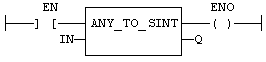# ANY_TO_SINT / ANY_TO_USINT

Operator - Converts the input into a small (8 bit) integer value.

## Inputs

IN : ANY  Input value

## Outputs

Q : SINT  Value converted to a small (8 bit) integer

## Remarks

For BOOL input data types, the output is 0 or 1. For REAL input data type, the output is the integer part of the input real. For TIME input data types, the result is the number of milliseconds. For STRING inputs, the output is the number represented by the string, or 0 if the string does not represent a valid number. In LD language, the conversion is executed only if the input rung (EN) is TRUE. The output rung (ENO) keeps the same value as the input rung. In IL Language, the ANY_TO_SINT function converts the current result.

## ST Language

Q := ANY_TO_SINT (IN);

## LD Language

(* The conversion is executed only if EN is TRUE *)
(* ENO keeps the same value as EN *)IL Language:

Op1: LD  IN
ANY_TO_SINT
ST  Q